Next: 5.4 Sensitivity of the Up: 5. Detection and Observation Previous: 5.2 Detection and Observation   Contents

# 5.3 Statistical Formulae

The problem of the detection/observation of an astronomical object superposed on a smooth sky background and imaged with a CCD detector can be mathematically described as the general problem of the detection/observation of a Poisson signal (photoelectrons originating from the object under consideration) in presence of Poisson noise (photoelectrons originating from the sky background) and Gaussian noise (readnoise). Following a simple statistical approach, general analytical formulae for the signal-to-noise ratios and standard errors of CCD photometry can be derived, which are convenient to use during the instrument design and mission planning phases because they give very similar errors to those obtained from simulations. The use of analytical formulae has the additional advantage over simulations that the relative importance of the various error sources is more easily seen.

Our goal is to derive general formulae expressing the signal-to-noise ratio produced by an object within a given aperture during a given exposure time, and then to apply them to the cases of detection and observation. As for detection, the estimation of the signal-to-noise ratio leads to estimate the number of galaxies that could be detected under the present assumptions for sampling and readnoise in the ASM1. As for observation, a comparison between the estimated accuracies in surface photometry achievable with different sample sizes can be given.

The conventions used in the following are as follows:

•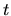: single-scan exposure time [s]
• aperture : the angular size of the sky area over which the measurement is performed [solid angle]
•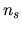: number of samples within the aperture
•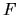: total electron counts within the aperture during[e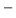]
•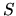: electron counts from the object within the aperture during[e]
•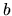: electron counts from the sky background per sample during[e]
•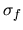: standard error of generic estimated flux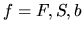[e]
•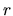: total readnoise per sample [erms]
•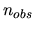: number of superposed observations
•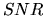: signal-to-noise ratio in the measurement of•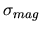: relative standard error in the measurement of[mag]
Under these assumptions,can be written as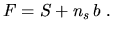(5.1)

The physical process of the emission of photons fron an astronomical object can be statistically described in terms of a Poisson distribution. The standard error in the measurement ofis then due to the intrinsic Poisson noise associated withand to the readnoise. These two contributions sum quadratically yielding for the variance of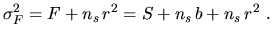(5.2)

Since the signalfrom the object is estimated by subtraction fromof the sky background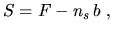(5.3)

the variance ofis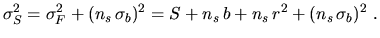(5.4)is then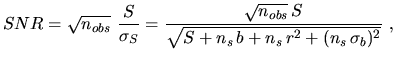(5.5)

whileis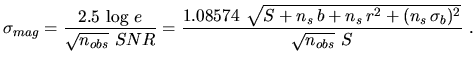(5.6)

Even thoughis given by Equation 5.5 both for detection and for observation, its meaning is very different in the two cases. In the case of detection, an highmeans a safe detection, i.e. an high chance of having detected a galaxy. As for observation, an highmeans an accurate observation, i.e. a small error in surface photometry. In order to apply the previous formulae, one needs to estimate the sensitivity, the readnoise and the sky background levels of the Astros.Next: 5.4 Sensitivity of the Up: 5. Detection and Observation Previous: 5.2 Detection and Observation   Contents
Mattia Vaccari 2000-12-05